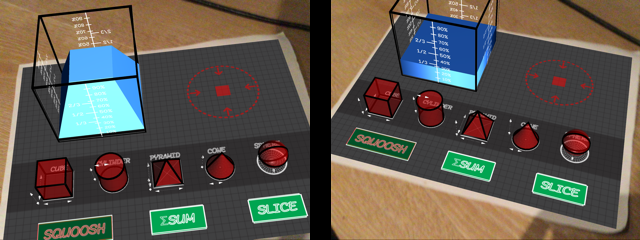## Squoosh Sum Slice Volumes

This module addresses Common Core 8.G.C.9

This module can be used individually or in conjunction with another textbook or existing curriculum. The magical graph paper itself can be used as a cheat sheet for deriving the volumes analytically.

Open-Ended Plan: This is a general guide that outlines the components of this module while referencing the magical graph paper.

Each volume is derived on the paper using non-calculus methods, appropriate for the middle school level. The cube and cylinder's volumes extend directly from the area of each slice. The pyramid's volume can be deduced by slicing a cube up into 6 equilateral pyramid's. The pyramid we want is actually twice. Once the pyramid volume is derived, it may be appropriate to pre-discuss Cavaliari's principle in equivalances of volumes, before using that to derive the cone volume. Finally, the volume of a sphere is derived by plastering on its surface area a bunch of pyramids spiking out from a locus, or imagining a "pyramid-porcupine." (We "explain away" the surface area as an unwrapped paper of the right ansatz proportions.)

## Unit Derivations

Plug in unit parameters to derive the r-notation used below (and on the magical graph paper), where r=1 is the radius of a unit sphere:
• Cube:
• Cylinder:
• Pyramid:
• Cone:
• Sphere:
Sample Lab: One suggested use is as a lab session where students discover these 3D objects and prove these volume formulae at the middle school level. This sample Lab Worksheet can be used for students to fill out as they discover more about each volume.

### Squoosh Lab

1. Squoosh each object.
2. Measure each volume ratio for each object and record it in the worksheet.
3. Note the percentage of a cube that is represented.
4. Discuss why each object is only a certain fraction of the cube's volume.
This can be used in conjunction with plugging in unit parameters in each volume formula, letting each dimension of the cube be equal to 2r: . Divide each unit volumes by this number to get the a priori ratio.When a pyramid with the same base area as the cube is melted in the cube beaker, it fills up only about one third of the cube's volume.

### Sum Lab

1. Select each object and change each slice's ΔH by moving the slider.
2. Record each volume in each corresponding ΔH.
3. Verify that as ΔH approaches 0, we get closer to the volume formula for each object.
4. Discuss why the volumes for each shape either decrease or increase with smaller slice ΔH values.

### Slice Lab

[Under Development] Drills - students can see 3D augmented reality versions of everyday objects such as soda cans and baseball's and calculate their volumes.

Last updated: May 6, 2015

#### Resources

Please see the Modules section for details on how each module works. See the Squoosh Sum Slice Volumes Module here.